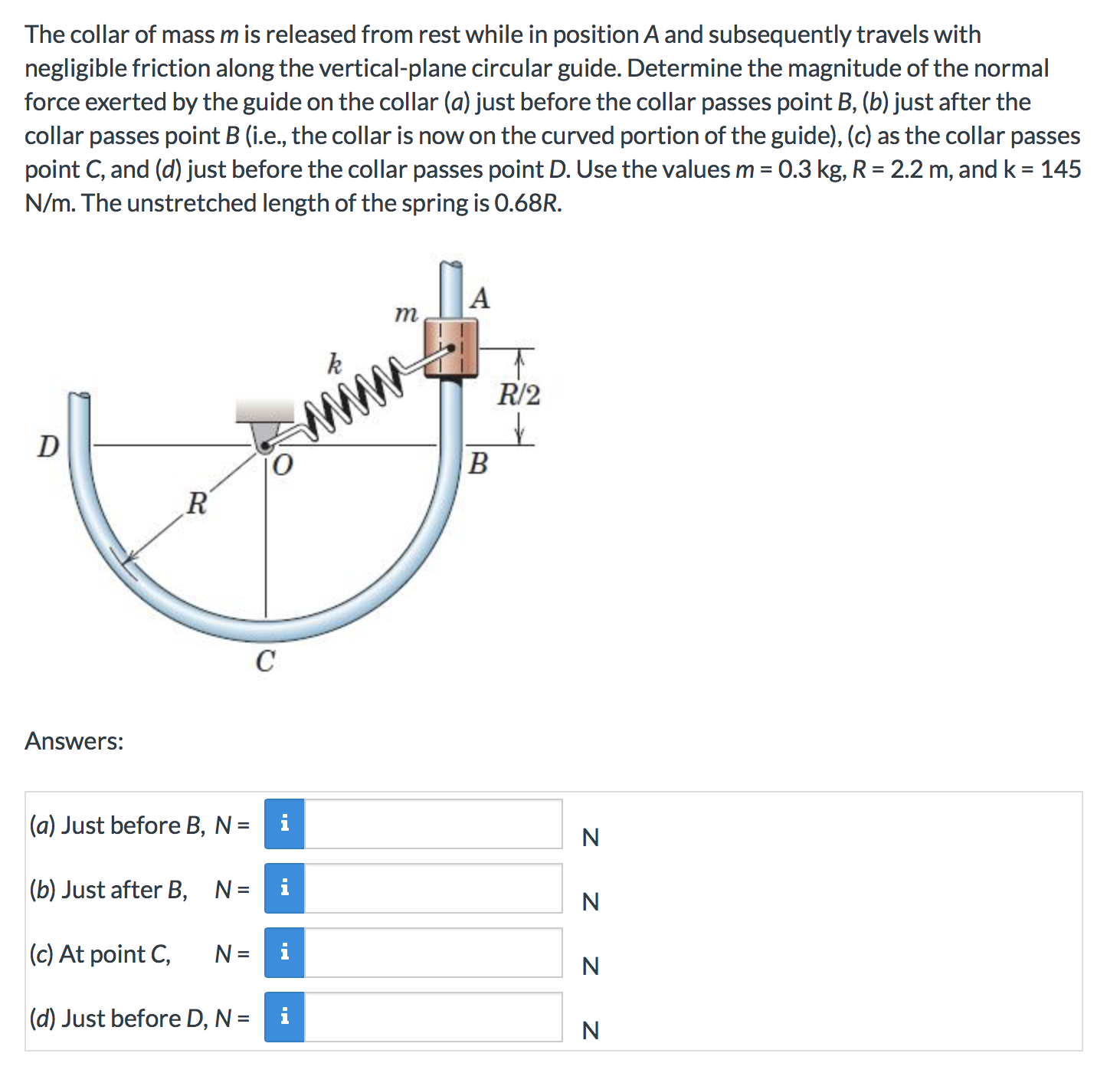# The collar of mass m is released from rest while in position A and subsequently travels with negligible friction along the vertical-plane circular guide. Determine the magnitude of the normal force exerted by the guide on the collar (a) just before the collar passes point B, (b) just after the collar passes point B (i.e., the collar is now on the curved portion of the guide), (c) as the collar passes point C, and (d) just before the collar passes point D. Use the values m 0.3 kg, R 2.2 m, and k 145 N/m. The unstretched length of the spring is 0.68R. = m R/2 www D R C Answers: (a) Just before B, N =i N |(b) Just after B, N= i (c) At point C i N (d) Just before D, N =i N z z z z

Questionhelp_outlineImage TranscriptioncloseThe collar of mass m is released from rest while in position A and subsequently travels with negligible friction along the vertical-plane circular guide. Determine the magnitude of the normal force exerted by the guide on the collar (a) just before the collar passes point B, (b) just after the collar passes point B (i.e., the collar is now on the curved portion of the guide), (c) as the collar passes point C, and (d) just before the collar passes point D. Use the values m 0.3 kg, R 2.2 m, and k 145 N/m. The unstretched length of the spring is 0.68R. = m R/2 www D R C Answers: (a) Just before B, N =i N |(b) Just after B, N= i (c) At point C i N (d) Just before D, N =i N z z z z fullscreen